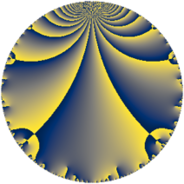# Properties

 Label 154.4.aLevel $154$ Weight $4$ Character orbit 154.a Rep. character $\chi_{154}(1,\cdot)$ Character field $\Q$ Dimension $14$ Newform subspaces $9$ Sturm bound $96$ Trace bound $3$

# Related objects

## Defining parameters

 Level: $$N$$ $$=$$ $$154 = 2 \cdot 7 \cdot 11$$ Weight: $$k$$ $$=$$ $$4$$ Character orbit: $$[\chi]$$ $$=$$ 154.a (trivial) Character field: $$\Q$$ Newform subspaces: $$9$$ Sturm bound: $$96$$ Trace bound: $$3$$ Distinguishing $$T_p$$: $$3$$

## Dimensions

The following table gives the dimensions of various subspaces of $$M_{4}(\Gamma_0(154))$$.

Total New Old
Modular forms 76 14 62
Cusp forms 68 14 54
Eisenstein series 8 0 8

The following table gives the dimensions of the cuspidal new subspaces with specified eigenvalues for the Atkin-Lehner operators and the Fricke involution.

$$2$$$$7$$$$11$$FrickeDim.
$$+$$$$+$$$$+$$$$+$$$$1$$
$$+$$$$+$$$$-$$$$-$$$$1$$
$$+$$$$-$$$$+$$$$-$$$$2$$
$$+$$$$-$$$$-$$$$+$$$$2$$
$$-$$$$+$$$$+$$$$-$$$$2$$
$$-$$$$+$$$$-$$$$+$$$$3$$
$$-$$$$-$$$$+$$$$+$$$$3$$
Plus space$$+$$$$9$$
Minus space$$-$$$$5$$

## Trace form

 $$14 q + 4 q^{2} - 8 q^{3} + 56 q^{4} + 36 q^{5} + 16 q^{8} + 154 q^{9} + O(q^{10})$$ $$14 q + 4 q^{2} - 8 q^{3} + 56 q^{4} + 36 q^{5} + 16 q^{8} + 154 q^{9} - 8 q^{10} - 22 q^{11} - 32 q^{12} + 76 q^{13} - 56 q^{14} + 52 q^{15} + 224 q^{16} - 60 q^{17} + 164 q^{18} - 80 q^{19} + 144 q^{20} - 44 q^{22} + 100 q^{23} + 246 q^{25} - 112 q^{26} - 68 q^{27} + 140 q^{29} + 272 q^{30} - 52 q^{31} + 64 q^{32} + 352 q^{33} + 680 q^{34} + 112 q^{35} + 616 q^{36} + 232 q^{37} - 168 q^{38} - 728 q^{39} - 32 q^{40} - 1196 q^{41} - 168 q^{42} - 280 q^{43} - 88 q^{44} - 736 q^{45} - 320 q^{46} - 504 q^{47} - 128 q^{48} + 686 q^{49} + 1452 q^{50} - 2024 q^{51} + 304 q^{52} - 596 q^{53} - 1200 q^{54} + 792 q^{55} - 224 q^{56} - 2240 q^{57} + 1496 q^{58} + 880 q^{59} + 208 q^{60} + 548 q^{61} - 688 q^{62} + 840 q^{63} + 896 q^{64} + 1120 q^{65} - 84 q^{67} - 240 q^{68} - 652 q^{69} - 280 q^{70} - 1508 q^{71} + 656 q^{72} + 468 q^{73} + 728 q^{74} + 308 q^{75} - 320 q^{76} - 308 q^{77} + 1264 q^{78} - 2552 q^{79} + 576 q^{80} + 1454 q^{81} - 3224 q^{82} - 3664 q^{83} - 3616 q^{85} - 736 q^{86} - 2936 q^{87} - 176 q^{88} + 3088 q^{89} - 2456 q^{90} + 476 q^{91} + 400 q^{92} - 68 q^{93} - 1520 q^{94} + 1232 q^{95} - 608 q^{97} + 196 q^{98} - 154 q^{99} + O(q^{100})$$

## Decomposition of $$S_{4}^{\mathrm{new}}(\Gamma_0(154))$$ into newform subspaces

Label Dim $A$ Field CM Traces A-L signs $q$-expansion
$a_{2}$ $a_{3}$ $a_{5}$ $a_{7}$ 2 7 11
154.4.a.a $1$ $9.086$ $$\Q$$ None $$-2$$ $$-5$$ $$-1$$ $$-7$$ $+$ $+$ $+$ $$q-2q^{2}-5q^{3}+4q^{4}-q^{5}+10q^{6}+\cdots$$
154.4.a.b $1$ $9.086$ $$\Q$$ None $$-2$$ $$0$$ $$2$$ $$-7$$ $+$ $+$ $-$ $$q-2q^{2}+4q^{4}+2q^{5}-7q^{7}-8q^{8}+\cdots$$
154.4.a.c $1$ $9.086$ $$\Q$$ None $$2$$ $$-10$$ $$-14$$ $$7$$ $-$ $-$ $+$ $$q+2q^{2}-10q^{3}+4q^{4}-14q^{5}-20q^{6}+\cdots$$
154.4.a.d $1$ $9.086$ $$\Q$$ None $$2$$ $$-2$$ $$18$$ $$7$$ $-$ $-$ $+$ $$q+2q^{2}-2q^{3}+4q^{4}+18q^{5}-4q^{6}+\cdots$$
154.4.a.e $1$ $9.086$ $$\Q$$ None $$2$$ $$7$$ $$3$$ $$7$$ $-$ $-$ $+$ $$q+2q^{2}+7q^{3}+4q^{4}+3q^{5}+14q^{6}+\cdots$$
154.4.a.f $2$ $9.086$ $$\Q(\sqrt{137})$$ None $$-4$$ $$-5$$ $$-7$$ $$14$$ $+$ $-$ $+$ $$q-2q^{2}+(-2-\beta )q^{3}+4q^{4}+(-4+\cdots)q^{5}+\cdots$$
154.4.a.g $2$ $9.086$ $$\Q(\sqrt{37})$$ None $$-4$$ $$6$$ $$26$$ $$14$$ $+$ $-$ $-$ $$q-2q^{2}+(3+\beta )q^{3}+4q^{4}+(13-\beta )q^{5}+\cdots$$
154.4.a.h $2$ $9.086$ $$\Q(\sqrt{57})$$ None $$4$$ $$-5$$ $$-17$$ $$-14$$ $-$ $+$ $+$ $$q+2q^{2}+(-2-\beta )q^{3}+4q^{4}+(-10+\cdots)q^{5}+\cdots$$
154.4.a.i $3$ $9.086$ 3.3.7636.1 None $$6$$ $$6$$ $$26$$ $$-21$$ $-$ $+$ $-$ $$q+2q^{2}+(2-\beta _{1})q^{3}+4q^{4}+(9-\beta _{2})q^{5}+\cdots$$

## Decomposition of $$S_{4}^{\mathrm{old}}(\Gamma_0(154))$$ into lower level spaces

$$S_{4}^{\mathrm{old}}(\Gamma_0(154)) \cong$$ $$S_{4}^{\mathrm{new}}(\Gamma_0(7))$$$$^{\oplus 4}$$$$\oplus$$$$S_{4}^{\mathrm{new}}(\Gamma_0(11))$$$$^{\oplus 4}$$$$\oplus$$$$S_{4}^{\mathrm{new}}(\Gamma_0(14))$$$$^{\oplus 2}$$$$\oplus$$$$S_{4}^{\mathrm{new}}(\Gamma_0(22))$$$$^{\oplus 2}$$$$\oplus$$$$S_{4}^{\mathrm{new}}(\Gamma_0(77))$$$$^{\oplus 2}$$